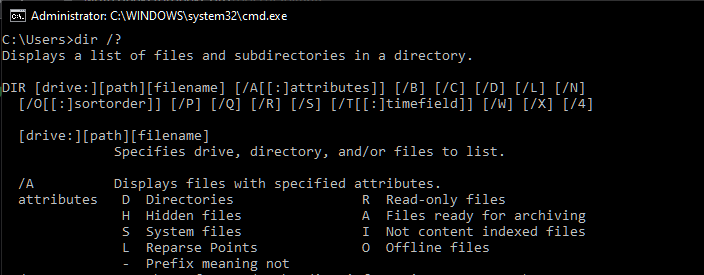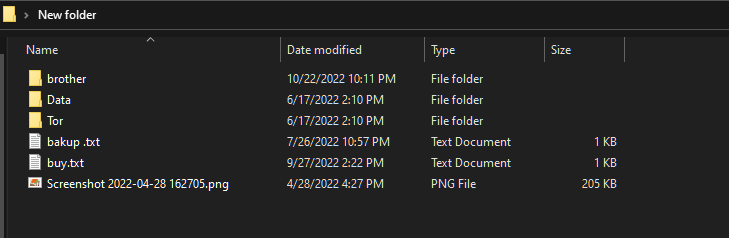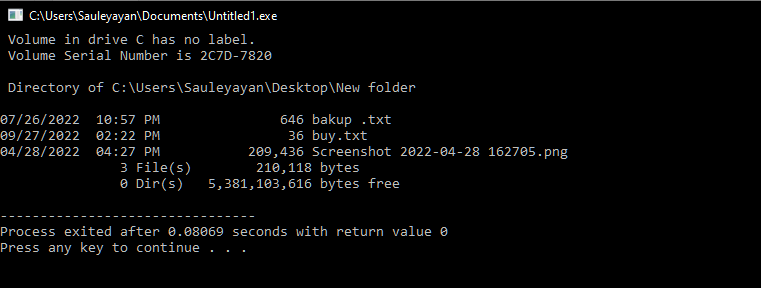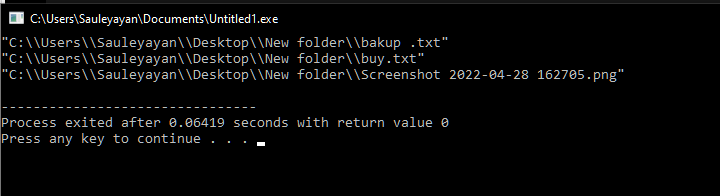Open in App
Not now

# C++ Program to Get the List of Files in a Directory

• Last Updated : 06 Feb, 2023

Getting the list of files in a directory is one of the most common operations performed by the Filesystem of an OS. The file explorer application in most operating systems performs the same operation in the background. In this article, you will learn how to get the list of files in a directory using C++.

Two methods can be used to Get the List of Files in a Directory:

• Using the system function  – ( functions in C++ )
• Using the directory_iterator – ( Invoking directory traversing commands from the operating system’s command interpreter )

## 1. Getting the list of files using the system function

The list of files in a directory could be obtained by calling the system function and sending an argument to dir /a-d for Windows OS (or ls -p | grep -v / in Linux OS). The help page of the command is as follows:Directory displayed using cmd

A Windows machine would be used for demonstration, and the list of files in the following directory would be obtained.File explorer to view directories

Code:

## C++

 `// C++ Program to Getting` `// the list of files using` `// the system function` `#include ` `#include ` `#include `   `using` `namespace` `std;`   `int` `main()` `{` `    ``// This variable contains the path to the directory` `    ``// The path should be given inside double quotes` `    ``// Therefore, escaped the enclosing quotes using` `    ``// backslash` `    ``string path(` `        ``"\"C:\\Users\\Sauleyayan\\Desktop\\New folder\""``);`   `    ``// The command which would do the file listing` `    ``// A space is added at the end of the command` `    ``// intentionally to make space for the path` `    ``string command(``"dir /a-d "``);`   `    ``// The path is appended after the command string` `    ``command.append(path);`   `    ``// The string is converted to const char * as system` `    ``// function requires` `    ``const` `char``* final_command = command.c_str();`   `    ``// Sending the final command as the argument to the` `    ``// system function` `    ``system``(final_command);`   `    ``return` `0;` `}`

Output:Output of Program

Explanation: Firstly, the cstdlib header file is imported to allow the use of the system function. Then the directory’s path on which the files are to be searched is assigned to the variable path. Then another variable named command is assigned the command to be run. After which, both variables are concatenated to produce the final command. Then another variable of type const char * is defined and assigned the value of the aforementioned concatenated string. Since the system function requires a const char * as an argument, this conversion was necessary. Then the char array (containing the command) is passed to the system function, which delivers the desired result.

## 2. Getting the list of files using the directory_iterator function

Getting the list of files within a directory could be done by using an iterator on the directory. Which will go through each element of the directory one by one. Then distinguishes whether the path belongs to a file or directory and, in the end, displays the valid ones.

The following code uses C++17 standard code.

Code:

## C++

 `// C++ Program for Getting` `// the list of files using` `// the directory_iterator function` `#include ` `#include ` `#include ` `#include `   `namespace` `fs = std::filesystem;`   `int` `main()` `{` `    ``// Path to the directory` `    ``std::string path` `        ``= ``"C:\\Users\\Sauleyayan\\Desktop\\New folder"``;`   `    ``// This structure would distinguish a file from a` `    ``// directory` `    ``struct` `stat sb;`   `    ``// Looping until all the items of the directory are` `    ``// exhausted` `    ``for` `(``const` `auto``& entry : fs::directory_iterator(path)) {`   `        ``// Converting the path to const char * in the` `        ``// subsequent lines` `        ``std::filesystem::path outfilename = entry.path();` `        ``std::string outfilename_str = outfilename.string();` `        ``const` `char``* path = outfilename_str.c_str();`   `        ``// Testing whether the path points to a` `        ``// non-directory or not If it does, displays path` `        ``if` `(stat(path, &sb) == 0 && !(sb.st_mode & S_IFDIR))` `            ``std ::cout << path << std::endl;` `    ``}` `}`

Output:Output of Program

Explanation: Firstly the header files filesystem and sys/stat.h were imported. Then a path to the directory is assigned to a variable. A structure template is initialized, which will be used to differentiate between a file and a directory. Then in a for loop, all the directory elements are iterated through. The first three statements in the for loop are just converting the path to the directory element from std::filesystem::path to const char * datatype. This is required as the stat function takes in an argument a character array, hence the requirement for this conversion. Then in an if the condition it is checked whether the path belongs to a directory or not. If it does not, then the path is displayed. Otherwise, it is ignored.

My Personal Notes arrow_drop_up
Related Articles